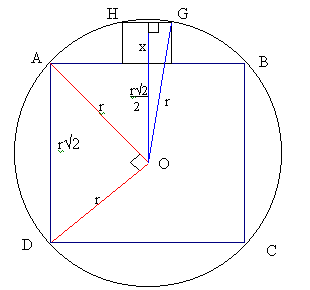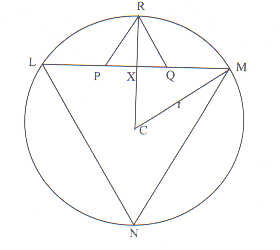#### You may also like### Doodles

Draw a 'doodle' - a closed intersecting curve drawn without taking pencil from paper. What can you prove about the intersections?### Russian Cubes

I want some cubes painted with three blue faces and three red faces. How many different cubes can be painted like that?### Picture Story

Can you see how this picture illustrates the formula for the sum of the first six cube numbers?

# Fitting In

##### Age 14 to 16 Challenge Level:

Well done Freddie Manners (Packwood Haugh School) for this solution of the first part of the problem. Perhaps someone else can solve the second part.

If the radius of the circle is $r$, the length of each side of the square $r\sqrt{2}$ (Pythagoras' theorem).

Take a triangle from centre of circle to mid-point $HG$, mid-point $HG$ to $G$, and $G$ to centre. This is a right-angled triangle.If the length of the sides of the small square is $x$, the sides of this triangle are:

$x/2$, $x + r\sqrt{2}/2$ and $r$.

By pythagoras, ${({1\over 2}x)^2} + {( {r\sqrt2\over2} + {x})^2} = {r^2}$ ${x^2\over4} + {2r^2\over4} + {xr\sqrt2} + {x^2} = {r^2}$ ${x^2} + {2r^2} + {4xr\sqrt2} + {4x^2} = {4r^2}$ ${5x^2} + {4xr\sqrt2} = {2r^2}$ Substitute $y = r\sqrt{2}$ (length of the side of the large square)

${5x^2} + {4xy} = {y^2}$ ${5x^2} + {4xy} - {y^2} ={ 0}$ ${x} = {{-4y \pm\sqrt{{(4y)^2} + {20y^2}}}\over 10}$ ${x} = {{-4y \pm\sqrt{36y^2}} \over 10}$ ${x} = {{-4y \pm 6y}\over 10}$ ${10x} = {2y or -10y}$

Ratio must be positive, therefore $10x = 2y$, therefore $y = 5x$
QED

Solution to part B.

Submitted by Samantha Gooneratne, Colombo International School, Sri Lanka. Well done Samantha! Her teacher also solved the problem using similar triangles, which I have include below Samantha's solution.

Let $X$ be the mid point of $PQ$, $C$ the center of the circle and $r$ the radius.

 $RX + XC = r$ Hence $PR \sin 60^{\circ}+ CM \cos 60^{\circ}=r$ ${{\sqrt{3}\over 2}+ {{1 \over2}r} = {r}}$ Therefore ${ {\sqrt{3}.PQ = {r}}}$ Now ${{LM} ={2(r \sin 60)}}$ so ${{LM} ={\sqrt{3}.r}}$ ${{LM} = {\sqrt{3}.(\sqrt{3}.PQ)}}$ ${{LM} = {3PQ}}$Solution using similar triangles:

$NR$ is a diameter so $NLR = 90^{\circ}$
but $NLM = 60^{\circ}$ so $RLP = 30^{\circ}$
Now $LPR = 120^{\circ}$ so $LRP = 30^{\circ}$
Hence $LP = PR$ but $PR = PQ$
so $LP = PQ = QM$
$LM = 3 PQ$